# Introduction to PWM – Pulse width modulation

In this article we will see an introduction of pulse width modulation. Pulse width modulation is also known as PWM. Pulse width modulation has many application in digital communication , power electronics, auto intensity control of street lights, speed control of dc motor and variable pwm to generate analog signal from digital signals, digital to analog converter.  There are many other applications of this technique. Two methods are used to generate PWM signals digital method and analog method. I will discuss both method at the end of this article.  so let’s start with basic introduction of pulse width modulation pulse width modulation or PWM is a technique for getting analog results with digital means. It also has applications in inverters, dc to dc power supplies.

### what is pulse width modulation

What you can do with it PWM? It stands for pulse width modulation and it consists of producing a square wave. you can control the up or high time in a PWM signal.  The minimum and maximum voltage are the values that limit the waves oscillation the space between them is called amplitude.  A cycle is the interval of the wave where you can find one full repetition the time a cycle takes to finish is called time period. Time period of a signal is

`Time period = on time + off time`

### Frequency of PWM

The frequency is 1 over a period which gives you how many cycles are in a time unit.  For example if the timer period of a signal is 20ms, its frequency will be 50Hz where Hz is unit of frequency. It is read a hertz.

`Frequency =  1 / timer period`

Picture below shows the amplitude and timer period of a wave form.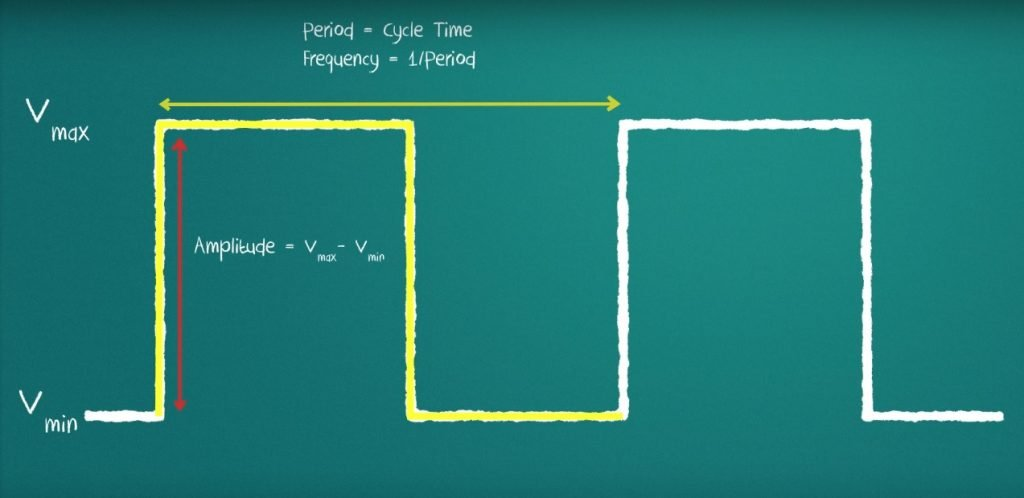### What is duty cycle ?

Duty Cycle is a important concept used in pulse width modulation. The duty cycle represents how much of the time  in which the signal is high in total time period.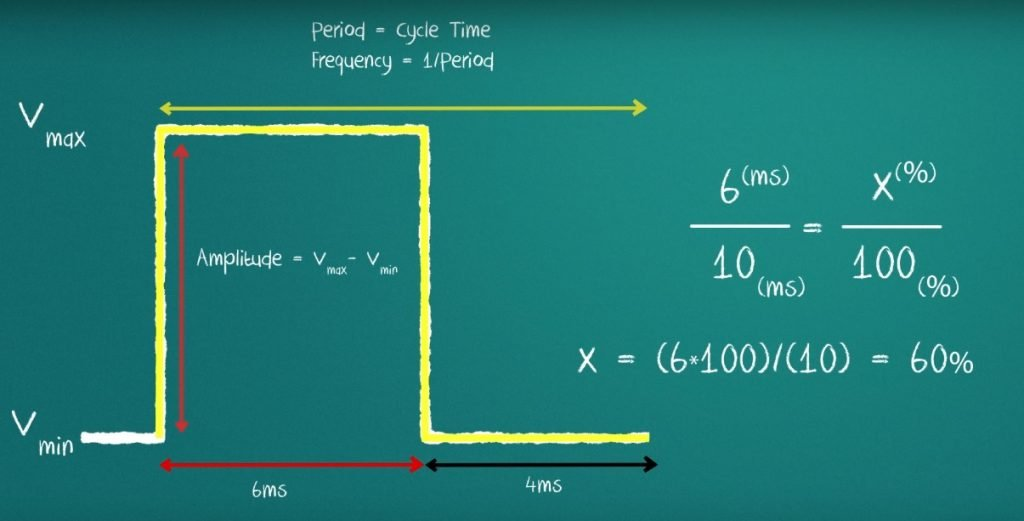So the formula for duty cycle is shown in following expression:

`Duty Cycle = (  On time of signal /  total timer period of signal )`

### How to calculate output voltage of PWM signal?

Now lets see how to calculate a duty cycle. To calculate duty cycle,  you need to know how much of the period the signal is high. Let us set our high time as 6 milliseconds and our low time as 4 milliseconds.  Total time period is 10ms. Now let’s use a simple rule to calculate the percentage of the period in which the signal is high relates it to total time period.

` Duty cycle =  6ms / 10ms  = 0.6`

By solving this we get the duty cycle of 0.6 which unitless quantity.  We always measure duty cycle in percentage. Maximum duty cycle can be 1 or 100% when on time or high time of signal is equal to total time period of signal. Similarly minimum duty cycle willbe  0 or 0% when signal is off for a total timer period. you can see a picture below of signals with different duty cycles.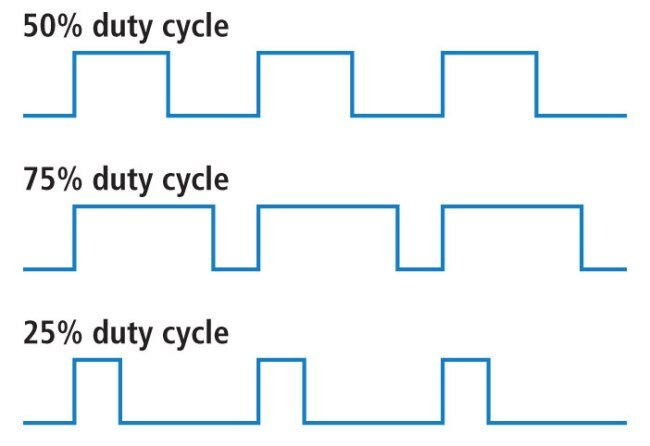### Amplitude of PWM

Amplitude of pulse width modulation is another important concept to discuss to fully understand this concept. Amplitude is a difference between a maximum voltage and minimum voltage of signal.

`Amplitude =  Vmax - Vmin`

In case of digital signals, minimum voltage is mostly zero. So amplitude is peak voltage of signal. let’s imagine a PWM signal that oscillates between 0 and 5 volts.  let’s say this signal has a duty cycle of 50%.  Something interesting will happen to the output voltage instead of being 5 volts is expected.  It will now be 2.5 volts simply.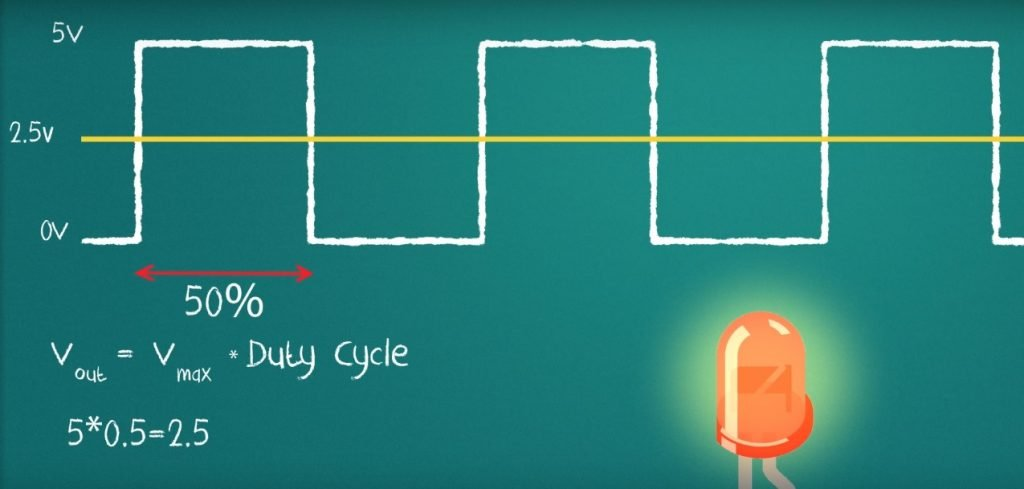When you apply this square wave of 50% duty cycle to an LED, you will get a voltage of 2.5 across the Light emitting diode.  Because when you apply a square wave of 50% duty cycle as a input voltage source, the formula of output voltage is given by :

`Vout = D x Vmax`

Where D is duty cycle of pulse with modulation signal or square wave. We multiply the max voltage by the duty cycle. According to above formula of output voltage, we can clearly see a direct relation between output voltage and duty cycle. The maximum voltage or amplitude of signal remains constant. To get higher voltage we need a higher duty cycle signal. To get lower voltage at the output, we need apply a lower duty cycle signal.

```Maximum output voltage will be equal = Vmax   when duty cycle = 100% or 1

Maximum output voltage will be equal = 0   when duty cycle = 0```

Similar concept is used in street lights to control intensity of street lights. Same method is used to in power electronics circuits to step down voltages using buck converter circuits.

So now you have learnt basic concepts of pulse width modulation like duty cycle,  on time of signal, off time of signal, time period of PWM and its amplitude. In rest of this article, I will explain different methods used to generate PWM signals.

### Pulse width modulation generation techniques

Two method are used to generate PWM:

1. PWM generation using digital circuits like Microcontroller.
2. PWM generation using analog circuit like operational amplifiers and comparator circuits.

### Pulse width modulation generation using microcontroller

To generate a digital signal with variable duty cycle, it is always recommend to use a microcontroller like Arduino. Because these microcontrollers have built in module available to generate digital signals. You can easily set duty cycle with the help of microcontroller programming in c.  I have already posted article on these concepts. Check these posts to generate PWM using a microcontroller.

### Pulse width modulation generation using analog circuits

The simplest way to generate PWM signal is using a operational amplifier. To generate a digital signal with operational amplifier, we use operational amplifier as a comparator circuit. Operational amplifier consists of two terminals non-inverting terminal and inverting terminal. We apply triangular wave at the non inverting input and control voltage input at the inverting pin of operational amplifier. Picture below show the whole processes of generating pwm signal using operational amplifier.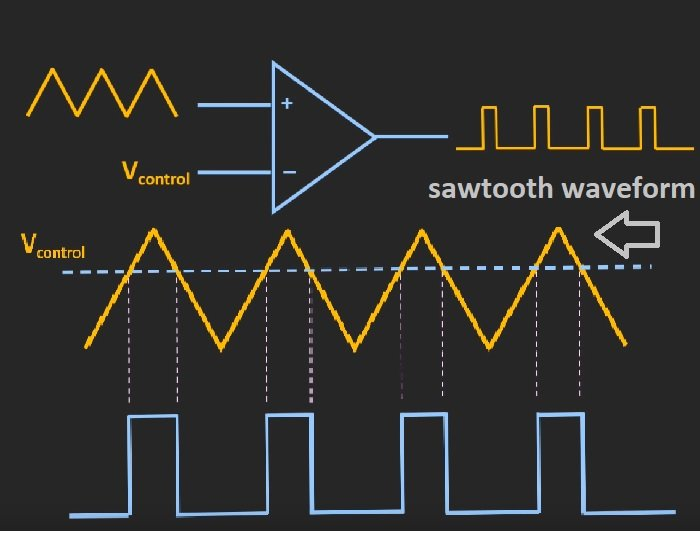So to understand working of this method, first you need to understand working of operational amplifier as a comparator circuit. So as you can understand from above pictures, when the triangular voltage applied to the non inverting input is less than the control voltage which is applied to inverting pin of operational amplifier,  output of comparator circuit will be low and whenever the voltage of triangular signal is greater than the control voltage, output of comparator will be high. So the on time of digital signal or pulse width modulation depends on the magnitude of control voltage voltage. So its mean control voltage and duty cycle is inversely proportional to each other.  Because duty cycle is directly proportional to on time of PWM signal. To get higher duty cycle, we need to decrease the value of control voltage. To get lower duty cycle, we need to increase the value of control voltage. So this is how easy it is to generate PWM with the help of analog electronics components.

### Other method to generate PWM

There are many integrated circuit available in market which are use to produce PWM signals and they also have ability to generate variable duty cycle digital signals. Name of some of them are given below:

### Applications of Pulse width modulation

I have mentioned few application at the start of this article. But you can my project in which I used PWM for various applications like inverters, three phase inverter and power electronics.